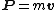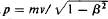momentum

(redirected from momentums)
Also found in: Dictionary, Thesaurus, Medical, Legal, Financial.

momentum

(mōmĕn`təm), in mechanics, the quantity of motion of a body, specifically the product of the mass of the body and its velocityvelocity,
change in displacement with respect to time. Displacement is the vector counterpart of distance, having both magnitude and direction. Velocity is therefore also a vector quantity. The magnitude of velocity is known as the speed of a body.
. Momentum is a vector quantity; i.e., it has both a magnitude and a direction, the direction being the same as that of the velocity vector. When an external force acts upon a body or a system of bodies in motion, it causes a change in the momentum of the body. The impulse of a force acting on a body is the product of the force and the duration of time in which it acts and is equal to the change in momentum of the body. When no external force acts upon a body in motion or a system of bodies there is no change in the total momentum even though, as in the case of a system of bodies, there may be an internal disturbance of the system resulting in changes in the momenta of individual bodies. This conclusion is commonly known as the principle of the conservation of momentum (see conservation lawsconservation laws,
in physics, basic laws that together determine which processes can or cannot occur in nature; each law maintains that the total value of the quantity governed by that law, e.g., mass or energy, remains unchanged during physical processes.
, in physics). The momentum of a body should not be confused with its kinetic energyenergy,
in physics, the ability or capacity to do work or to produce change. Forms of energy include heat, light, sound, electricity, and chemical energy. Energy and work are measured in the same units—foot-pounds, joules, ergs, or some other, depending on the system of
. The distinction between them can be seen in the action of a pile driver. The distance to which the pile is driven depends upon its kinetic energy; the length of time required for the action to cease, upon its momentum. In addition to the momentum a body has because of its linear motion, the body may also have angular momentum because of rotation. The angular momentum of a particle rotating about a point is equal to the product of the mass of the particle, its angular velocity, and the square of its distance from the axis of rotation. More simply, the angular momentum is the product of the instantaneous linear momentum and the distance. Angular momentum is a vector quantity directed perpendicular to the plane of motion.

Momentum

Linear momentum is the product of the mass and the linear velocity of a body. It is defined by Eq. (1),

(1)where m is the mass and v is the linear velocity. Since linear momentum is the product of a scalar and a vector quantity, it is a vector and hence has both magnitude and direction.

According to the general statement of Newton's second law, for a force F , a momentum P , and a time t, Eq. (2) holds.

(2)Thus Newton's second law involves the time rate of change of momentum. Changes of momentum are important in collision processes. See Collision (physics)

When a group of bodies is subject only to forces that members of the group exert on one another, the total momentum of the group remains constant. See Angular momentum, Conservation of momentum, Impulse (mechanics)

momentum

1. (linear momentum) Symbol: p. A property of a body moving in a straight line. It is equal in magnitude to the product of the mass and velocity of the body. It is a vector quantity, mv , having the same direction as the velocity. See also conservation of momentum; Newton's laws of motion.

Momentum

a measure of mechanical motion that for a mass point is equal to the product of the mass m of the point and its velocity v. Momentum, mv, is a vector quantity, with the same direction as the velocity of the point. Upon the action of a force, the momentum of the point changes, usually both numerically and in direction. This change is determined by the second (basic) law of dynamics.

The momentum Q of a mechanical system is equal to the geometric sum of the momentums of all its points or the product of the mass M of the entire system and the velocity vc of its center of mass: Q = Σ mkvk = Mvc. A change in the momentum of the system takes place only through the action of external forces—that is, forces acting on the system from bodies that are not part of the system. According to the theory of the change of momentum, Q1 – Q0 = ΣSke, where Q0 and Q1 are the momentums of the system at the beginning and end of a certain time interval and Ske are the momentums of external forces Fke over the time interval (this theorem is expressed in differential form by the equation dQ/dt = ΣFke). This theorem is used for the solution of many problems in dynamics, particularly in impact theory.

For a closed system (that is, for a system that does not experience external forces), or in the case in which the geometric sum of the external forces acting on the system equals zero, the law of conservation of momentum is valid. In such cases the momentums of the individual parts of the system (for example, during the action of internal forces) may change, but in such a way that the quantity Q = Σ mkvk remains constant. This law explains such phenomena as jet propulsion, the recoil upon firing a gun, and the operation of a screw propeller or oars. For example, if a gun and bullet are regarded as a single system, then the pressure of the powder gases upon firing will be an internal force for the system and cannot change the momentum of the system, which was equal to zero before the firing. Therefore, in imparting the momentum m1v1 to the bullet in the direction toward the muzzle, the powder gases simultaneously impart to the gun a momentum m2v2, which is numerically equal but opposite in direction, resulting in the recoil. If the velocity v1 of the bullet upon its exit from the barrel is known, the maximum recoil velocity v2 may be found from the equation m1v1 = m2v2 (Where v1 and v2 are scalar quantities of velocity).

At velocities close to the speed of light c, the momentum of a free particle is given by the formula, where β = v/c. When ν << c, this formula becomes the ordinary formula p = mv.

Momentum exists for physical fields (electromagnetic, gravitational, and so on). The momentum of a field is characterized by momentum density (the relationship of the momentum of a unit volume to the entire volume) and is expressed as field intensity or field potential.

S. M. TARG

momentum

[mō′ment·əm]
(mechanics)
Also known as linear momentum; vector momentum.
For a single nonrelativistic particle, the product of the mass and the velocity of a particle.
For a single relativistic particle, m v/(1 -v 2/ c 2)1/2, where m is the rest-mass,vthe velocity, and c the speed of light.
For a system of particles, the vector sum of the momenta (as in the first or second definition) of the particles.

momentum

Of a moving body, the product of the mass of the body and its velocity.

momentum

1. Physics the product of a body's mass and its velocity.
2. the impetus of a body resulting from its motion
References in periodicals archive ?
For exactly this reason, more than 325 operators, including 18 of the top 50, have chosen to partner with Momentum.
Okay, that last line may be a bit of a generalization because while I was happy overall with the way the Momentums reproduced music, there were a couple of areas where it fell short.
As is seen no notion about the Abraham or Minkowski momentums has been used.
As an exhibitor at one of the largest annual events for the channel partner community, Momentum is poised to become the talk of the show with their innovative Mobility View application along with sneak peaks of their newly redesigned and highly anticipated subscriber portal that is slated to be released later this year.
In one of the nations fastest growing marketplaces, Momentum has been enabling partners to reach commercial hosted voice growth of 40% across their customer base.
With the changing landscape of the cable industry, the ACA Summit is a special opportunity for operators to discover a new revenue flow with a proven leader they already know and trust in Momentum Telecom.
Momentum Telecom will utilize the investment to further accelerate its already tremendous growth in the cloud communications space through acquisitions, new product offerings and investments in internal teams.
Momentum is thrilled to be showcasing the ability of their hosted voice solution to transform cooperatives and ignite a new path to revenue.
As Calix expands into new markets and assists cable operators with their transition to fiber deep architectures and their deployment of PON, working with experienced partners like Momentum Telecom is crucial to our success, said John Czeban, area vice president of MSO sales at Calix.
Cramer joins the Momentum team with more than 18 years of experience in the telecommunications industry.
Momentum works with their customers and agents to create a fully integrated system to fit the needs of any business.
The two organizations commemorated the occasion with a small ribbon cutting ceremony attended by Momentum Telecom representatives along with several TWO MEN AND A TRUCK employees including Jake Gaitan, IT Director.

Site: Follow: Share:
Open / Close